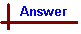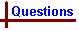States and Properties of Matter - The Gas Laws (2) Your feedback on these self-help problems is appreciated. Click here to send an e-mail. For on-line help with this topic, see the chemcal module "Behaviour of Gases". Shortcut to Questions Q: 1 2 3 4 5 1 The density of a gas at 298 K and 101 kPa is 1.70 gram litre-1. Calculate its molar mass.2 An unknown compound is analysed and its empirical formula determined to be CHCl. The vapour density measured at 380 K and 101 kPa is 3.10 gram litre-1. What is the molecular formula?3 A fluorocarbon has the molecular formula C4F8. Calculate the density of this gas at 400 K and 202 kPa?4 At what temperature will the density of methane gas be 0.65 gram litre-1 if the pressure is 101 kPa?5 The density of a certain gas is 1.375 gram litre-1 at 273 K and 101.3 kPa. Calculate its density at 303 K and 98.0 kPa.The Gas Laws 2 (Answers) 1 The problems in this section combine the Ideal Gas Equation (IGE) with the definition of a mole = mass / molar mass. PV = nRT = (mass / molar mass)RT The density is 1.70 g litre-1 Take a volume of 1.00 L Therefore, mass = 1.70 g. P = 101 kPa, T = 298 K and moles, n, = 1.70 / molar mass Substitute in the IGE 101 x 1.00 = (1.70 / molar mass) x 8.314 x 298 [Note: for P given in kPa and V in L, R has the value 8.314 J K-1 mol-1.T is always in Kelvin.] molar mass = (1.70 x 8.314 x 298) / (101 x 1.00) = 41.7 g mol-12 The empirical formula is CHCl, so its empirical formula weight = 48.5 PV = nRT = (mass / molar mass)RT The density is 3.10 g litre-1 Take a volume of 1.00 L Therefore, mass = 3.10 g Substitute in the IGE: P = 101 kPa, T = 380 K and moles, n, = (3.10 / molar mass) 101 x 1.00 = (3.10 / molar mass) x 8.314 x 380 molar mass = (3.10 x 8.314 x 380) / (101 x 1.00) = 97.0 g mol-1 Therefore molar weight = 97.0 = 2 x empirical formula weight and molecular formula is C2H2Cl2.3 The molar mass of C4F8 = 200.0 g mol-1. PV = nRT = (mass / molar mass)RT Take 1.00 L of the gas. Let m = the mass of the gas present in 1.00 L at 400 K and 202 kPa. Therefore moles of gas at these conditions = m / molar mass = m / 200.0 mol. The density at these conditions = mass / V = (m / 1.00) g L-1 To calculate m and thence density, substitute in the Ideal Gas Equation with P = 202 kPa and T = 400 K: 202 x 1.00 = (m / 200.0) x 8.314 x 400 m = (202 x 1.00 x 200) / (8.314 x 400) = 12.1 g As V = 1.00 L, therefore density = 12.1 g L-1.4 The molar mass of methane, CH4, = 16.05 g mol-1 Density = 0.65 g L-1, so for a volume = 1.00 L, mass = 0.65 g, and number of moles in 1.00 L = 0.65 / 16.05 = 0.04050 mol PV = nRT 101 x 1.00 = 0.04050 x 8.314 x T Therefore, T = (101 x 1.00) / (0.04050 x 8.314) = 300 K [Note: T is always in Kelvin in the Ideal Gas Equation]5 From the first set of data, the molar mass of the gas can be calculated: P = 101.3 kPa, T = 273 K, d = 1.375 g L-1 Therefore, for V = 1.00 L, mass of gas = 1.375 g Let MW be the molar mass of the gas Then number of moles present = n = (1.375 / MW) Substitute in the ideal gas equation: 101.3 x 1.00 = (1.375 / MW) x 8.313 x 273 Therefore MW = (1.375 x 8.314 x 273) / (101.3 x 1.00) = 30.8 Using this result, the mass (m) of 1.00 L of the gas at the new conditions can be deduced by again using the IGE: P = 98.0 kPa, T = 303 K, moles of gas, n, = (m / 30.8) 98.0 x 1.00 = (m / 30.8) x 8.314 x 303 m = (98.0 x 1.00 x 30.8) / (8.314 x 303) = 1.199 g As this is the mass of gas in 1.00 L, then density = m / V = 1.199 g L-1.